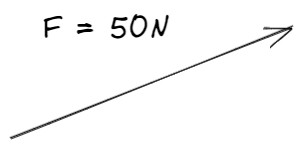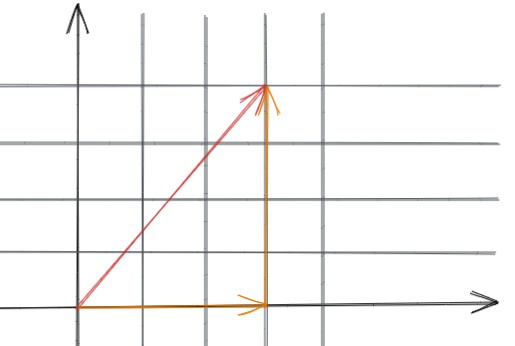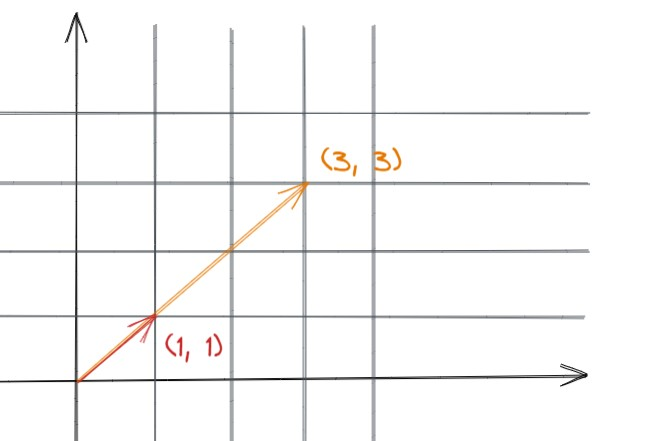# 向量

## 向量是什么？## 向量的加法和减法

``````(x1, y1, ...) + (x1, y1, ...) = (x1 + x2, y1 + y2, ...)
```````(0, 0)`点直接移动到`(3, 4)`点，与先从`(0, 0)`点移动到`(3, 0)`点，再移动到`(3, 4)`点的结果没有区别，所以有以下等式成立。

``````// 等式左边表示直接从(0, 0)点移动到(3, 4)点
// 等式右边表示先从(0, 0)点移动到(3, 0)点，再从(3, 0)点移动到(3, 4)点
(3, 4) = (3, 0) + (0, 4)
``````

``````(x1, y1, ...) - (x1, y1, ...) = (x1 - x2, y1 - y2, ...)
``````

## 向量的数乘

``````scalar * (x1, y1, ...) = (x1 * scalar, y1 * scalar, ....)
``````